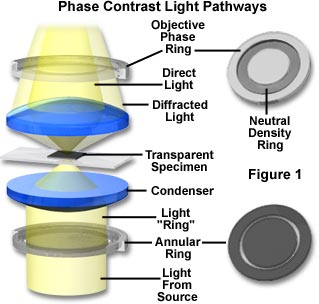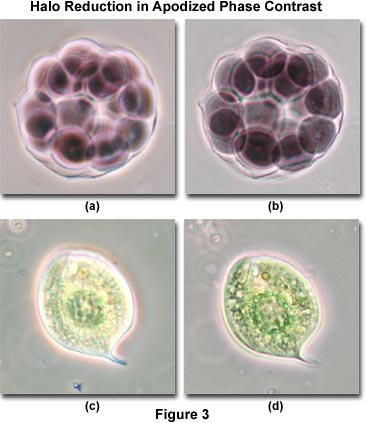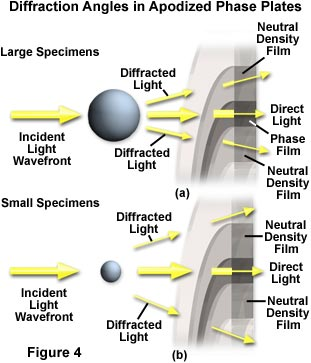# 研究尼康显微镜减少光晕与变迹相衬的效果

2016-04-05

光的吸收差异往往是在活细胞内的各种细胞内的成分和质膜之间可以忽略不计，使他们几乎不可见的观察时，利用明场照明的经典技术的显微镜。相差显微镜利用微小的折射率的差异在细胞成分和未染色的细胞和其周围的水溶液中，在这些和类似的透明标本产生的对比。(1)

δ = (2Π/λ)(n' - n)d

(2)

r = 0.61λ/n(sin(Θ))

(3)

r = d = 0.61λ/n(sin(θ))

(4)

sin(θ) = 0.61λ/nd

(5)

sin(θ) = (2π/λ)(n' - n)0.61λ/nδ

(6)

sin(θ) = 2π(n' - n)0.61/nδ

(7)

sin(θ) ∝ 1/δ

(8)

φ(0) = sin(ωt)

(9)

φ(1) = sin(ωt + δ)

(10)

φ(1) = sin(ωt) + δcos(ωt)

（11）

φ（1）=（1 +δ）cos（ωT）

(12)

I = (1 + δ)2